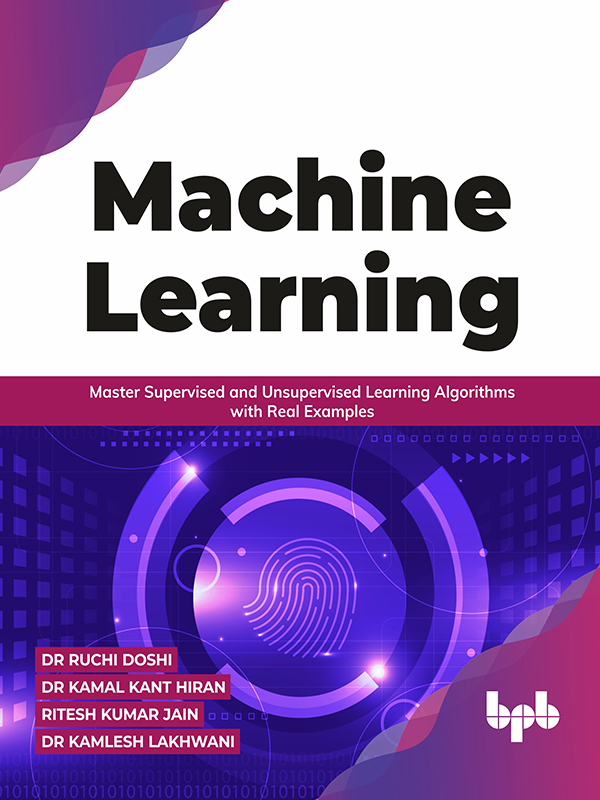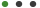# Machine Learning

Master Supervised and Unsupervised Learning Algorithms with Real Examples

Formats - PDF, EPUB

Pages - 296,      ISBN - 9789391392352

Language - English

Published on 12/2021#### Description

Concepts of Machine Learning with Practical Approaches.

## Key Features

● Includes real-scenario examples to explain the working of Machine Learning algorithms.
● Includes graphical and statistical representation to simplify modeling Machine Learning and Neural Networks.
● Full of Python codes, numerous exercises, and model question papers for data science students.

## Description

The book offers the readers the fundamental concepts of Machine Learning techniques in a user-friendly language. The book aims to give in-depth knowledge of the different Machine Learning (ML) algorithms and the practical implementation of the various ML approaches.

This book covers different Supervised Machine Learning algorithms such as Linear Regression Model, Naïve Bayes classifier Decision Tree, K-nearest neighbor, Logistic Regression, Support Vector Machine, Random forest algorithms, Unsupervised Machine Learning algorithms such as k-means clustering, Hierarchical Clustering, Probabilistic clustering, Association rule mining, Apriori Algorithm, f-p growth algorithm, Gaussian mixture model and Reinforcement Learning algorithm such as Markov Decision Process (MDP), Bellman equations, policy evaluation using Monte Carlo, Policy iteration and Value iteration, Q-Learning, State-Action-Reward-State-Action (SARSA). It also includes various feature extraction and feature selection techniques, the Recommender System, and a brief overview of Deep Learning.

By the end of this book, the reader can understand Machine Learning concepts and easily implement various ML algorithms to real-world problems.

## What you will learn

● Perform feature extraction and feature selection techniques.
● Learn to select the best Machine Learning algorithm for a given problem.
● Get a stronghold in using popular Python libraries like Scikit-learn, pandas, and matplotlib.
● Practice how to implement different types of Machine Learning techniques.

## Who this book is for

This book is designed for data science and analytics students, academicians, and researchers who want to explore the concepts of machine learning and practice the understanding of real cases. Knowing basic statistical and programming concepts would be good, although not mandatory.